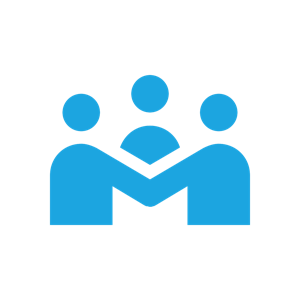# Algebra 2 IntroductionGrade-Level Mathematics Actions and Processes Descriptions of the Mathematics Actions and Processes provide a sense of what students are doing as they develop into mathematically literate students.Suggested Learning Progression (v2) This year-long progression provides a sample vision for the learner experience that engages in meaningful, connected mathematics.Objective Analysis Analysis for each grade-level objective is provided in a manner to support deep understanding for the teacher.Engagement Strategies Educators can engage their students in math activities using these strategies.

## An Introduction to Algebra 2

The mathematics learning experience in Algebra 2 builds on the students’ prior experiences of functions in Algebra 1.  Students in this course will develop a sophisticated mathematical understanding of the world around them as they interpret real-world phenomena through functions.  They will persevere through problem-solving processes and communicate their procedures and results mathematically.  Students will achieve procedural fluency resulting from conceptual understanding of the four major strands: Numbers & Operations, including complex numbers; Algebraic Reasoning & Algebra, solving nonlinear equations and using them to solve real-world problems; Functions, performing operations with functions, inverse functions, and interpreting characteristics of functions and their graphs; and Data & Probability.  Algebra 2 is a springboard to upper level math courses and career paths.

Introduction to the OKMath Framework

Algebra 2 Introduction